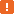# Creating Object PropertiesTo implement a property on a custom runtime object, you need to create the property “get” and “set” methods. The get method is executed when the property is read; the set method - when the property is assigned a new value. To track the actual property value, you can use a global script variable, to which the get and set methods will refer. The point in providing access to the property via the get and set methods is that these methods can not only return or assign the property value, but also perform some additional actions. For example, validate the value type before assigning the property, convert the value to the needed type and format, and so on.

The access type of the property -- read-write, read-only or write-only -- depends which of get and set methods it has:

• If the property has both get and set methods, it is considered read-write.
• If the property has only the get method, it is considered read-only.
• If the property has only the set method, it is considered write-only.

The get and set methods can have arbitrary names, since the correspondence between the property and its get and set methods is defined in the description.xml file. However, it is recommended that the get method has the `GetPropertyName`, `Get_PropertyName` or similar name, and the set method is named `SetPropertyName`, `Set_PropertyName` in a similar way. In this case, it is easier to understand and maintain the object’s source code.

If you wish to implement an ordinary property (that is, a property that has no parameters), the property’s get method must have no parameters. As for the set method, it must have one parameter, which corresponds to the new property value.

The following example demonstrates the source code of a sample read-write property that can hold string values:

JScript

// script.js

var Text;

// Returns the property value
function GetText()
{
return Text;
}

// Sets the property value
function SetText(Value)
{
Text = aqConvert.VarToStr(Value);
}

VBScript

' script.vbs

Dim Text

' Returns the property value
Function GetText
GetText = Text
End Function

' Sets the property value
Sub SetText(Value)
Text = aqConvert.VarToStr(Value)
End Sub

It is also possible to create indexed (parameterized) properties. These properties provide access to different values when different parameter values are used. The get method of an indexed property must have the same number of parameters as the property is supposed to take. The set method, in its turn, must have the same number of parameters as the property has, plus an additional parameter - the value to be assigned to the property. This parameter must be the last one in the set method declaration.

Below is the source code of a sample indexed property that provides access to array elements:

JScript

// script.js

var arr = new Array (1, 2, 3, 4, 5);

// Returns an array element specified by index
function GetItem(Index)
{
if ((Index < 0) || (Index >= arr.length))
{
Log.Error(aqString.Format("Index out of range: %d. The total number of items: %d", Index, arr.length));
return null;
}
else
return arr[Index];
}

// Sets the value of an array element specified by index
function SetItem(Index, Value)
{
if ((Index < 0) || (Index >= arr.length))
Log.Error(aqString.Format("Index out of range: %d. The total number of items: %d", Index, arr.length))
else
arr[Index] = Value;
}

VBScript

' script.vbs

arr = Array (1, 2, 3, 4, 5)

' Returns an array element specified by index
Function GetItem(Index)
If Index < 0 Or Index > UBound(arr) Then
Log.Error(aqString.Format("Index out of range: %d. The total number of items: %d", Index, UBound(arr)))
GetItem = Null
Else
GetItem = arr(Index)
End If
End Function

' Sets the value of an array element specified by index
Sub SetItem(Index, Value)
If Index < 0 Or Index > UBound(arr) Then
Log.Error(aqString.Format("Index out of range: %d. The total number of items: %d", Index, UBound(arr)))
Else
arr(Index) = Value
End If
End SubMake sure to implement the parameter type and range checking in the get and set methods of indexed properties. Invalid index values and types are among the most frequent scripting errors. To learn how you can handle these errors, see Handling Errors in Script Extensions.